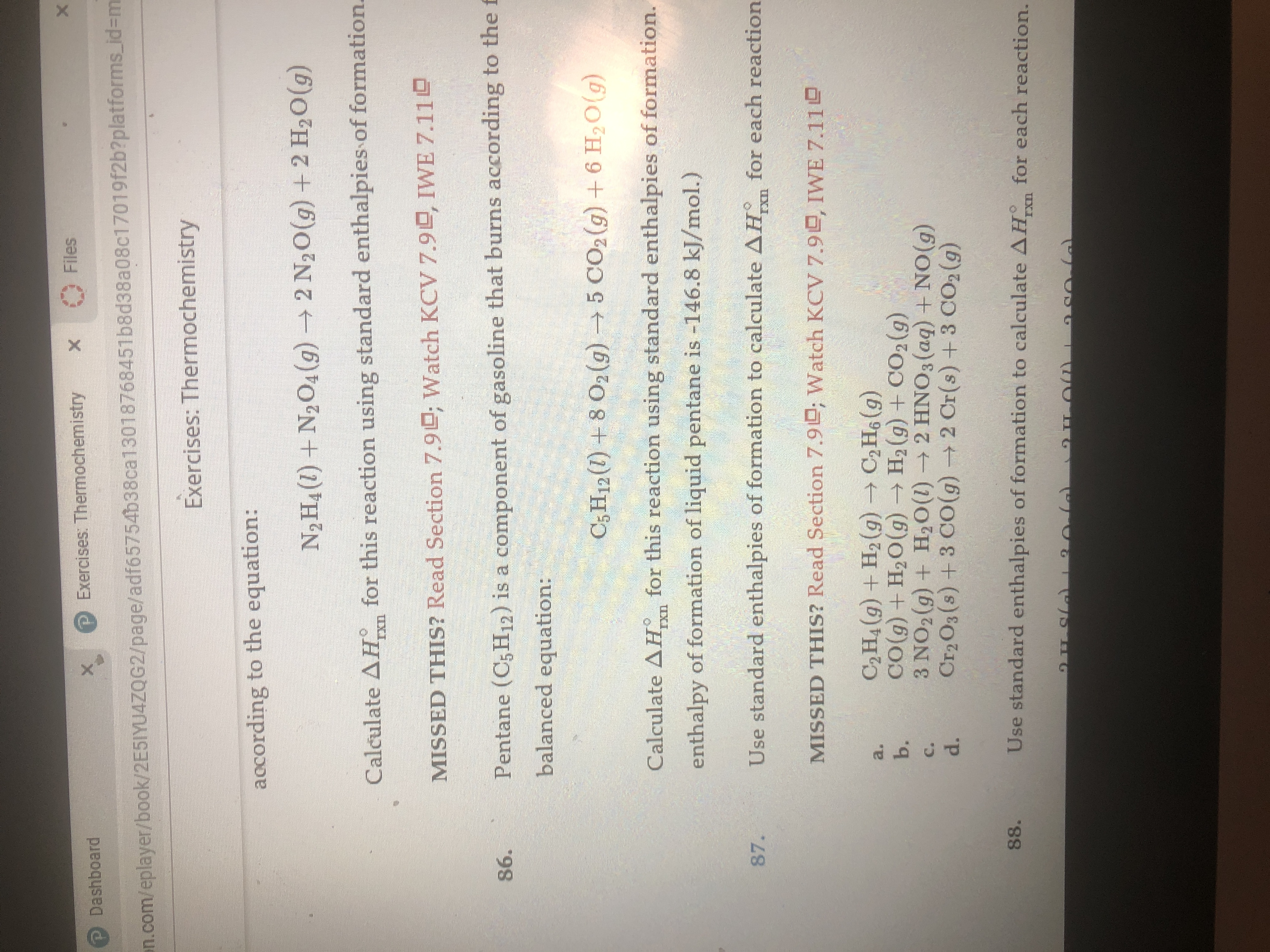# XFilesXExercises: ThermochemistryXP Dashboardn.com/eplayer/book/2E5IYU4ZQG2/page/adf65754b38ca13018768451b8d38a08c17019f2b?platforms.id=mExercises: Thermochemistryaocording to the equation:N2 H4 (1)+N204(g)2 N2O(g) + 2 H20(g)Calculate AH for this reaction using standard enthalpies of formation.IXDWatch KCV 7.9L, IWE 7.11LMISSED THIS? Read Section 7.9Pentane (C5 H12) is a component of gasoline that burns according to the f86.balanced equation:C5H12 (1)8 02 (g)5 CO2 (g) +6 H20(g)for this reaction using standard enthalpies of formation.Calculate AHenthalpy of formation of liquid pentane is -146.8 kJ/mol.)Use standard enthalpies of formation to calculate AH for each reaction87.IXnMISSED THIS? Read Section 7.9; Watch KCV 7.9D, IWE 7.11C2H4(g) +H2 (g) C2H6 (g)CO(g) + H2O(g)H2 (g) +CO2(g)3 NO2(g) + H2O (I)2 HNO3(aq) +NO(g)Cr2O3(s)+3 CO (g)2 Cr(s) +3 CO2 (g)a.b.C.d.Use standard enthalpies of formation to calculate AH for each reaction.88.IXn

Question
8 views

How do I complete #87?help_outlineImage TranscriptioncloseX Files X Exercises: Thermochemistry X P Dashboard n.com/eplayer/book/2E5IYU4ZQG2/page/adf65754b38ca13018768451b8d38a08c17019f2b?platforms.id=m Exercises: Thermochemistry aocording to the equation: N2 H4 (1)+N204(g)2 N2O(g) + 2 H20(g) Calculate AH for this reaction using standard enthalpies of formation. IXD Watch KCV 7.9L, IWE 7.11L MISSED THIS? Read Section 7.9 Pentane (C5 H12) is a component of gasoline that burns according to the f 86. balanced equation: C5H12 (1)8 02 (g)5 CO2 (g) +6 H20(g) for this reaction using standard enthalpies of formation. Calculate AH enthalpy of formation of liquid pentane is -146.8 kJ/mol.) Use standard enthalpies of formation to calculate AH for each reaction 87. IXn MISSED THIS? Read Section 7.9; Watch KCV 7.9D, IWE 7.11 C2H4(g) +H2 (g) C2H6 (g) CO(g) + H2O(g)H2 (g) +CO2(g) 3 NO2(g) + H2O (I)2 HNO3(aq) +NO(g) Cr2O3(s)+3 CO (g)2 Cr(s) +3 CO2 (g) a. b. C. d. Use standard enthalpies of formation to calculate AH for each reaction. 88. IXn fullscreen
check_circle

Step 1

The heat of a reaction can be calculated by knowing the standard enthalpies of formation of reactants and products.

Step 2

The balanced required equation is given below.

Step 3

The value of standard enthalpies of formation of...

### Want to see the full answer?

See Solution

#### Want to see this answer and more?

Solutions are written by subject experts who are available 24/7. Questions are typically answered within 1 hour.*

See Solution
*Response times may vary by subject and question.
Tagged in

### Physical Chemistry